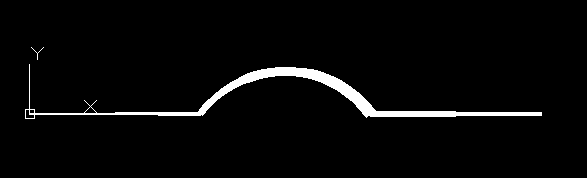# Tutorial for LWPolyline¶

The `LWPolyline` is defined as a single graphic entity, which differs from the old-style `Polyline` entity, which is defined as a group of sub-entities. `LWPolyline` display faster (in AutoCAD) and consume less disk space, it is a planar element, therefore all points in OCS as `(x, y)` tuples (`LWPolyline.dxf.elevation` is the z-axis value).

Create a simple polyline:

```import ezdxf

doc = ezdxf.new('R2000')
msp = doc.modelspace()

points = [(0, 0), (3, 0), (6, 3), (6, 6)]
msp.add_lwpolyline(points)

doc.saveas("lwpolyline1.dxf")
```

Append multiple points to a polyline:

```doc = ezdxf.readfile("lwpolyline1.dxf")
msp = doc.modelspace()

line = msp.query('LWPOLYLINE')  # take first LWPolyline
line.append_points([(8, 7), (10, 7)])

doc.saveas("lwpolyline2.dxf")
```

Getting points always returns a 5-tuple `(x, y, start_width, ent_width, bulge)`, start_width, end_width and bulge is `0` if not present:

```first_point = line
x, y, start_width, end_width, bulge = first_point
```

Use context manager to edit polyline points, this method was introduced because accessing single points was very slow, but since ezdxf v0.8.9, direct access by index operator `[]` is very fast and using the context manager is not required anymore. Advantage of the context manager is the ability to use a user defined point format:

```doc = ezdxf.readfile("lwpolyline2.dxf")
msp = doc.modelspace()

line = msp.query('LWPOLYLINE').first # take first LWPolyline, 'first' was introduced with v0.10

with line.points('xyseb') as points:
# points is a standard python list
# existing points are 5-tuples, but new points can be
# set as (x, y, [start_width, [end_width, [bulge]]]) tuple
# set start_width, end_width to 0 to be ignored (x, y, 0, 0, bulge).

del points[-2:]  # delete last 2 points
points.extend([(4, 7), (0, 7)])  # adding 2 other points
# the same as one command
# points[-2:] = [(4, 7), (0, 7)]

doc.saveas("lwpolyline3.dxf")
```

Each line segment can have a different start- and end-width, if omitted start- and end-width is `0`:

```doc = ezdxf.new('R2000')
msp = doc.modelspace()

# point format = (x, y, [start_width, [end_width, [bulge]]])
# set start_width, end_width to 0 to be ignored (x, y, 0, 0, bulge).

points = [(0, 0, .1, .15), (3, 0, .2, .25), (6, 3, .3, .35), (6, 6)]
msp.add_lwpolyline(points)

doc.saveas("lwpolyline4.dxf")
```

The first point carries the start- and end-width of the first segment, the second point of the second segment and so on, the start- and end-width value of the last point is used for the closing segment if polyline is closed else the values are ignored. Start- and end-width only works if the DXF attribute `dxf.const_width` is unset, to be sure delete it:

```del line.dxf.const_width # no exception will be raised if const_width is already unset
```

`LWPolyline` can also have curved elements, they are defined by the Bulge value:

```doc = ezdxf.new('R2000')
msp = doc.modelspace()

# point format = (x, y, [start_width, [end_width, [bulge]]])
# set start_width, end_width to 0 to be ignored (x, y, 0, 0, bulge).

points = [(0, 0, 0, .05), (3, 0, .1, .2, -.5), (6, 0, .1, .05), (9, 0)]
msp.add_lwpolyline(points)

doc.saveas("lwpolyline5.dxf")
```The curved segment is drawn from the point which defines the bulge value to the following point, the curved segment is always aa arc, The bulge value defines the ratio of the arc sagitta (segment height h) to half line segment length (point distance), a bulge value of `1` defines a semicircle. bulge > `0` the curve is on the right side of the vertex connection line, bulge < `0` the curve is on the left side.

ezdxf v0.8.9 supports a user defined points format, default is `xyseb`:

• `x` = x coordinate
• `y` = y coordinate
• `s` = start width
• `e` = end width
• `b` = bulge value
• `v` = (x, y) as tuple
```msp.add_lwpolyline([(0, 0, 0), (10, 0, 1), (20, 0, 0)], format='xyb')
msp.add_lwpolyline([(0, 10, 0), (10, 10, .5), (20, 10, 0)], format='xyb')
```